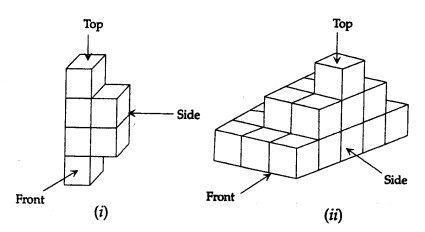## ML Aggarwal Class 7 Solutions for ICSE Maths Chapter 15 Visualising Solid Shapes Check Your Progress

Question 1.
Identify the nets which can be used to make a tetrahedron (cut out copies of the nets and try it):Solution:
The net/nets for a tetrahedron is (i) and (iii).

Question 2.
If four cubes each with 2 cm edge are placed side by side, what would the dimensions of the resultant cuboid be?
Solution:
Four cubes with 2 cm edge are placed side by side,
then the dimensions of the resulting cuboid will be
Length = 4 × 2 = 8 cm, breadth = 2 cm and height = 2 cm
An isometric sketch and oblique sketches are given below:Question 3.
Two dice are placed side by side as shown in the given figure. What would be the total on the face opposite to
(i) 5 + 6
(b) 4 + 3?Solution:
When two dice are placed side by side
as shown in the given figure,
the total would be on face opposite to
(i) 5 + 6 is 3
(ii) 4 + 7 is 7

Question 4.
For the structures given below sketch the front, side, and top view:Solution:
The front view side view and top view of the given two sketches are given below: